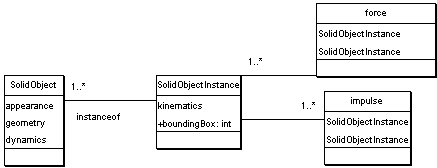# 3D Theory - Using a relational database to store 3d physics simulation

## Prerequisites

This page suggests a possible way to store the physics information. If you are not familiar with this subject you may like to look at the following pages first:

## Requirement for a flat file structure

When we are simulating physics, there may be a large number of moving objects and interactions, therefore it is imporant that we are able to process the information very fast. We have discussed the advantages of storing the information as a scenegraph here, however when we run the simulation a flat structure is more efficient because:

• Collision detection needs to be done between objects using the same coordinate system.
• When a force or impulse is acting between two objects we must be using the same frame-of-reference for the forces to be calculated correctly.
• We cannot apply Newtons laws in a rotating inertial frame.

So here is a possible file structure to hold the physics information.This diagram was generated by Argo, file is here if you want to edit it.

This model is divided into 2 large tables, the SolidObject definition and the SolidObject instance. In order to discuss this, take the example of a snooker or pool game, we may have an object to define a ball, the geometry and dynamics (mass and inertia tensor) are common to all the balls so this only has to be defined once. However the kinematics (position, velocity, etc.) is different for each ball, so each one has to be defined seperately which is why it is in a seperate table.

How can we take account of the different colour of the snooker balls, this implies that we need to put the appearance information (colour or texture) into the SolidObjectInstance table. This is fine for the snooker example, but in other examples the instances may differ in other ways, for instance a human animation may have different shapes but have the same basic structure. If we put all the parameters in the instance table then it would be massive.

So,

• What information do we store in the flat file structure?
• Do we store the information for every instance, or just the definition of the object?

Any ideas?

## Next Step and Further Reading

To see how these nodes could be used to implement a game goto this page